﻿ 对流扩散方程的非振荡守恒特征差分法 Non Oscillation Conservative Characteristic Difference Method for Solving Convection Diffusion Equations

Vol. 07  No. 11 ( 2018 ), Article ID: 27709 , 12 pages
10.12677/AAM.2018.711169

Non Oscillation Conservative Characteristic Difference Method for Solving Convection Diffusion Equations

Qianqian Wang, Lin Li, Yuqing Zhao, Zhongguo Zhou*

School of Information Science and Engineering, Shandong Agricultural University, Tai’an Shandong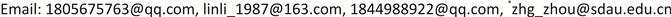Received: Oct. 28th, 2018; accepted: Nov. 19th, 2018; published: Nov. 26th, 2018ABSTRACT

The characteristic difference method based on quadratic Lagrange interpolation is used to solve the convection dominated diffusion problem, but there will be a larger numerical oscillation and not conservative. Combining the operator splitting, non-oscillatory and mass correction methods, the non-oscillation conservative characteristic difference methods are proposed to solve the convection diffusion equations. Firstly, the partial differential equations in two dimensions are splitting into two one-dimensional partial differential equations along the x-direction and the y-direction, respectively. Secondly, the second-order essentially non-oscillation and MMOCAA schemes are presented to compute the equations. By the numerical results, it shows that the scheme not only meets non-oscillatory and mass conservation, but also effectively solves the convection-dominant diffusion problems.

Keywords:Convection Diffusion Equations, Operator Splitting, Characteristic Difference Method, Essentially Non Oscillatory Interpolation, MMOCAA Scheme1. 引言

2. 模型

2.1. 对流扩散方程

$\left\{\begin{array}{l}\frac{\partial c}{\partial t}+\nabla \left(uc-D\nabla c\right)=f,\text{\hspace{0.17em}}\text{\hspace{0.17em}}\text{\hspace{0.17em}}\text{\hspace{0.17em}}\text{ }\left(x,y\right)\in \Omega ,\text{\hspace{0.17em}}\text{\hspace{0.17em}}t\in \left[0,T\right],\\ c\left(x,y,t\right)=0,\text{\hspace{0.17em}}\text{\hspace{0.17em}}\text{\hspace{0.17em}}\text{\hspace{0.17em}}\text{\hspace{0.17em}}\text{\hspace{0.17em}}\text{\hspace{0.17em}}\text{\hspace{0.17em}}\text{\hspace{0.17em}}\text{\hspace{0.17em}}\text{\hspace{0.17em}}\text{\hspace{0.17em}}\text{\hspace{0.17em}}\text{\hspace{0.17em}}\text{\hspace{0.17em}}\text{\hspace{0.17em}}\text{\hspace{0.17em}}\text{\hspace{0.17em}}\left(x,y\right)\in \partial \Omega ,\text{\hspace{0.17em}}\text{\hspace{0.17em}}t\in \left[0,T\right],\\ {c}_{0}\left(x,y\right)={c}_{0},\text{\hspace{0.17em}}\text{\hspace{0.17em}}\text{\hspace{0.17em}}\text{\hspace{0.17em}}\text{\hspace{0.17em}}\text{\hspace{0.17em}}\text{\hspace{0.17em}}\text{\hspace{0.17em}}\text{\hspace{0.17em}}\text{\hspace{0.17em}}\text{\hspace{0.17em}}\text{\hspace{0.17em}}\text{\hspace{0.17em}}\text{\hspace{0.17em}}\text{\hspace{0.17em}}\text{\hspace{0.17em}}\text{\hspace{0.17em}}\text{\hspace{0.17em}}\left(x,y\right)\in \Omega .\end{array}$ (2.1)

2.2. LOD算子分裂

$\left\{\begin{array}{l}\frac{\partial c}{\partial t}+\frac{\partial }{\partial x}\left({u}_{1}c-{D}_{1}\frac{\partial c}{\partial x}\right)=f\\ \frac{\partial c}{\partial t}+\frac{\partial }{\partial y}\left({u}_{2}c-{D}_{2}\frac{\partial c}{\partial y}\right)=0\end{array}$ (2.2)

3. 算法

3.1. 特征线法

Step 1：沿x方向

$\frac{\partial c}{\partial t}+\frac{\partial }{\partial x}\left({u}_{1}c-{D}_{1}\frac{\partial c}{\partial x}\right)=f,$ (3.1)

$\frac{\partial c}{\partial {\tau }_{1}}=\frac{\partial c}{\partial t}\mathrm{cos}\alpha +\frac{\partial c}{\partial x}\mathrm{cos}\beta .$ (3.2)

${\tau }_{1}$ 为x方向特征线， $\beta$$\alpha$ 分别为特征线与x和t方向的夹角，则

$\left\{\begin{array}{l}\mathrm{cos}\alpha =\frac{1}{\sqrt{1+{u}_{1}^{2}}}\\ \mathrm{cos}\beta =\frac{{u}_{1}}{\sqrt{1+{u}_{1}^{2}}}\end{array}$

$\frac{\partial c}{\partial t}\frac{1}{\sqrt{1+{u}_{1}^{2}}}+\frac{\partial c}{\partial x}\frac{{u}_{1}}{\sqrt{1+{u}_{1}^{2}}}=\frac{\partial c}{\partial {\tau }_{1}}.$

$\frac{\partial c}{\partial {\tau }_{1}}\sqrt{1+{u}_{1}^{2}}-{D}_{1}\frac{{\partial }^{2}c}{\partial {x}^{2}}=f,$

$\frac{{C}_{ij}^{n-\frac{1}{2}}-{\stackrel{¯}{C}}_{ij}^{n-1}}{\Delta t}-{D}_{1}\frac{{C}_{i\text{+}1，j}^{n-\frac{1}{2}}-2{C}_{ij}^{n-\frac{1}{2}}+{C}_{i-1,j}^{n-\frac{1}{2}}}{{h}^{2}}={f}_{ij}.\text{ }$ (3.3)

$\left\{{C}_{ij}\right\}$ 表示 $\left\{{c}_{ij}\right\}$ 的数值解， $\left\{{\stackrel{¯}{C}}_{ij}\right\}$ 将在后面给出。

Step 2：沿y方向

$\frac{\partial c}{\partial t}+\frac{\partial }{\partial y}\left({u}_{2}c-{D}_{2}\frac{\partial c}{\partial y}\right)=0,$

$\frac{\partial c}{\partial {\tau }_{2}}=\frac{\partial c}{\partial t}\mathrm{cos}\varphi +\frac{\partial c}{\partial y}\mathrm{cos}\phi ,$

$\frac{{C}_{ij}^{n}-{\stackrel{¯}{C}}_{ij}^{n-\frac{1}{2}}}{\Delta t}-{D}_{2}\frac{{C}_{i,j+1}^{n}-2{C}_{ij}^{n}+{C}_{i,j-1}^{n}}{{h}^{2}}=0,$

(1-a) 沿x方向，令

${\stackrel{¯}{C}}_{ij}^{n-1}={I}_{1}{C}_{ij}^{n-1}\left({\stackrel{¯}{x}}_{i}\right),{\lambda }_{1}=-\frac{{u}_{1}\Delta t}{h},{\stackrel{¯}{x}}_{i}={x}_{i}-{u}_{1}\Delta t.$

(1-b) 引入三个质量通量： $t={t}^{n-1}$ 时的初始质量通量

${R}^{n-1}={\sum }_{i=1}^{N}{\sum }_{j=1}^{N}{C}_{ij}^{n-1}{h}^{2}.$

${\stackrel{¯}{R}}^{n-1}={\sum }_{i=1}^{N}{\sum }_{j=1}^{N}{\stackrel{¯}{C}}_{ij}^{n-1}{h}^{2}.$

${\stackrel{˜}{R}}^{n-1}={\sum }_{i=1}^{N}{\sum }_{j=1}^{N}{\stackrel{˜}{C}}_{ij}^{n-1}{h}^{2}.$

${\stackrel{˜}{C}}_{ij}^{n-1}=\left\{\begin{array}{l}\mathrm{max}\left({I}_{1}{C}_{ij}^{n-1}\left({\stackrel{˜}{x}}_{i}^{+}\right),{I}_{1}{C}_{ij}^{n-1}\left({\stackrel{˜}{x}}_{i}^{-}\right)\right),{R}^{n-1}>{\stackrel{¯}{R}}^{n-1},\\ \mathrm{min}\left({I}_{1}{C}_{ij}^{n-1}\left({\stackrel{˜}{x}}_{i}^{+}\right),{I}_{1}{C}_{ij}^{n-1}\left({\stackrel{˜}{x}}_{i}^{-}\right)\right),{R}^{n-1}\le {\stackrel{¯}{R}}^{n-1},\end{array}$

${\stackrel{˜}{x}}_{i}^{+}={\stackrel{¯}{x}}_{i}+{\delta }_{1}$${\stackrel{˜}{x}}_{i}^{-}={\stackrel{¯}{x}}_{i}-{\delta }_{1}$${\delta }_{1}={r}_{1}{u}_{1}{\left(\Delta t\right)}^{2}$${r}_{1}=\frac{{D}_{1}\Delta t}{{h}^{2}}$$0<{r}_{1}<2$

(1-c) 质量扰动参数 ${\theta }_{1}$ 的确定

${R}^{n-1}={\theta }_{1}{\stackrel{¯}{R}}^{n-1}+\left(1-{\theta }_{1}\right){\stackrel{˜}{R}}^{n-1}.$

(1-d) 定义 $\left\{{\stackrel{^}{C}}_{ij}^{n-1}\right\}$

${\stackrel{^}{C}}_{ij}^{n-1}={\theta }_{1}{\stackrel{¯}{C}}_{ij}^{n-1}+\left(1-{\theta }_{1}\right){\stackrel{˜}{C}}_{ij}^{n-1}.$

(1-e) 计算 $\left\{{C}_{ij}^{n-\frac{1}{2}}\right\}$

$\frac{{C}_{ij}^{n-\frac{1}{2}}-{\stackrel{^}{C}}_{ij}^{n-1}}{\Delta t}-{D}_{1}\frac{{C}_{i+1,j}^{n-\frac{1}{2}}-2{C}_{ij}^{n-\frac{1}{2}}+{C}_{i-1,j}^{n-\frac{1}{2}}}{{h}^{2}}={f}_{ij}.\text{ }$ (3.4)

${C}_{ij}^{n-\frac{1}{2}}-{\stackrel{^}{C}}_{ij}^{n-1}-{r}_{1}\left({C}_{i+1,j}^{n-\frac{1}{2}}-2{C}_{ij}^{n-\frac{1}{2}}+{C}_{i-1,j}^{n-\frac{1}{2}}\right)={f}_{ij}\Delta t.$

$-{r}_{1}{C}_{i+1,j}^{n-\frac{1}{2}}+\left(2{r}_{1}+1\right){C}_{ij}^{n-\frac{1}{2}}-{r}_{1}{C}_{i-1,j}^{n-\frac{1}{2}}={f}_{ij}\Delta t+{\stackrel{^}{C}}_{ij}^{n-1}.\text{ }$ (3.5)

$\left(\begin{array}{ccccc}2{r}_{1}+1& -{r}_{1}& & & \\ -{r}_{1}& 2{r}_{1}+1& -{r}_{1}& & \\ & \ddots & \ddots & \ddots & \\ & & \ddots & \ddots & -{r}_{1}\\ & & & -{r}_{1}& 2{r}_{1}+1\end{array}\right)\left(\begin{array}{c}{c}_{1}\\ {c}_{2}\\ ⋮\\ {c}_{N-1}\\ {c}_{N}\end{array}\right)=\left(\begin{array}{c}{F}_{1}\\ {F}_{2}\\ ⋮\\ {F}_{N-1}\\ {F}_{N}\end{array}\right)$

$AC=F$

$A=\left(\begin{array}{ccccc}2{r}_{1}+1& -{r}_{1}& & & \\ -{r}_{1}& 2{r}_{1}+1& -{r}_{1}& & \\ & \ddots & \ddots & \ddots & \\ & & \ddots & \ddots & -{r}_{1}\\ & & & -{r}_{1}& 2{r}_{1}+1\end{array}\right)$

(2-a) 沿y方向，

${\stackrel{¯}{C}}_{ij}^{n-\frac{1}{2}}={I}_{2}{C}_{ij}^{n-\frac{1}{2}}\left({\stackrel{¯}{y}}_{j}\right),\text{ }\text{ }\text{ }\text{ }\text{ }{\lambda }_{2}=-\frac{{u}_{2}\Delta t}{h},$

(2-b) 质量通量。 $t={t}^{n-\frac{1}{2}}$ 时的质量通量

${R}^{n-\frac{1}{2}}={\sum }_{i=1}^{N}{\sum }_{j=1}^{N}{C}_{ij}^{n-\frac{1}{2}}{h}^{2}.$

${\stackrel{¯}{R}}^{n-\frac{1}{2}}={\sum }_{i=1}^{N}{\sum }_{j=1}^{N}{\stackrel{¯}{C}}_{ij}^{n-\frac{1}{2}}{h}^{2}.$

${\stackrel{˜}{R}}^{n-\frac{1}{2}}={\sum }_{i=1}^{N}{\sum }_{j=1}^{N}{\stackrel{˜}{C}}_{ij}^{n-\frac{1}{2}}{h}^{2}.$

${\stackrel{˜}{C}}_{ij}^{n-\frac{1}{2}}=\left\{\begin{array}{l}\mathrm{max}\left({I}_{2}{C}_{ij}^{n-\frac{1}{2}}\left({\stackrel{˜}{y}}_{j}^{+}\right),{I}_{2}{C}_{ij}^{n-\frac{1}{2}}\left({\stackrel{˜}{y}}_{j}^{-}\right)\right),\text{ }\text{ }\text{ }\text{ }\text{ }{R}^{n-\frac{1}{2}}>{\stackrel{¯}{R}}^{n-\frac{1}{2}},\\ \mathrm{min}\left({I}_{2}{C}_{ij}^{n-\frac{1}{2}}\left({\stackrel{˜}{y}}_{j}^{+}\right),{I}_{2}{C}_{ij}^{n-\frac{1}{2}}\left({\stackrel{˜}{y}}_{j}^{-}\right)\right),\text{ }\text{ }\text{ }\text{ }\text{ }{R}^{n-\frac{1}{2}}\le {\stackrel{¯}{R}}^{n-\frac{1}{2}},\end{array}$

${\stackrel{˜}{y}}_{j}^{+}={\stackrel{¯}{y}}_{j}+{\eta }_{2}$${\stackrel{˜}{y}}_{j}^{-}={\stackrel{¯}{y}}_{j}-{\eta }_{2}$ 其中， ${\eta }_{2}={r}_{2}{u}_{2}{\left(\Delta t\right)}^{2}$

(2-c) ${\theta }_{2}$ 的确定

${\stackrel{¯}{R}}^{n-\frac{1}{2}}\ne {\stackrel{˜}{R}}^{n-\frac{1}{2}}$ 时， ${\theta }_{2}$ 被确定为

${R}^{n-\frac{1}{2}}={\theta }_{2}{\stackrel{¯}{R}}^{n-\frac{1}{2}}+\left(1-{\theta }_{2}\right){\stackrel{˜}{R}}^{n-\frac{1}{2}}.$

(2-d) 计算 $\left\{{C}_{ij}^{n}\right\}$ 。定义 $\left\{{\stackrel{^}{C}}_{ij}^{n-\frac{1}{2}}\right\}$ 满足

${\stackrel{^}{C}}_{ij}^{n-\frac{1}{2}}={\theta }_{2}{\stackrel{¯}{C}}_{ij}^{n-\frac{1}{2}}+\left(1-{\theta }_{2}\right){\stackrel{˜}{C}}_{ij}^{n-\frac{1}{2}}.$

(3.4)式加入质量校正后，得

$\frac{{C}_{ij}^{n}-{\stackrel{^}{C}}_{ij}^{n-\frac{1}{2}}}{\Delta t}-{D}_{2}\frac{{C}_{i,j+1}^{n}-2{C}_{ij}^{n}+{C}_{i,j-1}^{n}}{{h}^{2}}=0,$ (3.6)

${r}_{2}=\frac{{D}_{2}\Delta t}{{h}^{2}}$ ，得

$-{r}_{2}{C}_{ij}^{n}+\left(2{r}_{2}+1\right){C}_{ij}^{n}-{r}_{2}{C}_{i,j-1}^{n}={\stackrel{^}{C}}_{ij}^{n-\frac{1}{2}}.\text{ }$ (3.7)

$BC=F$

3.2. 本质非振荡插值

1) 当 ${u}_{1}$ 为正数时，

(1-1) 当 $|{C}_{i-2,j}^{n-1}-2{C}_{i-1,j}^{n-1}+{C}_{ij}^{n-1}|>|{C}_{i+1,j}^{n-1}-2{C}_{ij}^{n-1}+{C}_{i-1,j}^{n-1}|$ 时，利用牛顿插值得

$\begin{array}{c}{\stackrel{¯}{C}}_{ij}^{n-1}={C}_{ij}^{n-1}+\frac{{C}_{ij}^{n-1}-{C}_{i-1,j}^{n-1}}{h}\left({\stackrel{¯}{x}}_{i}-{x}_{i}\right)+\frac{{C}_{i+1,j}^{n-1}-2{C}_{ij}^{n-1}+{C}_{i-1,j}^{n-1}}{2{h}^{2}}\left({\stackrel{¯}{x}}_{i}-{x}_{i-1}\right)\left({\stackrel{¯}{x}}_{i}-{x}_{i}\right)\\ =\frac{1}{2{h}^{2}}\left({\stackrel{¯}{x}}_{i}-{x}_{i-1}\right)\left({\stackrel{¯}{x}}_{i}-{x}_{i}\right){C}_{i+1,j}^{n-1}+\left[1+\frac{1}{h}\left({\stackrel{¯}{x}}_{i}-{x}_{i}\right)-\frac{1}{{h}^{2}}\left({\stackrel{¯}{x}}_{i}-{x}_{i-1}\right)\left({\stackrel{¯}{x}}_{i}-{x}_{i}\right)\right]{C}_{ij}^{n-1}\\ \text{\hspace{0.17em}}\text{\hspace{0.17em}}+\left[-\frac{1}{h}\left({\stackrel{¯}{x}}_{i}-{x}_{i}\right)+\frac{1}{2{h}^{2}}\left({\stackrel{¯}{x}}_{i}-{x}_{i-1}\right)\left({\stackrel{¯}{x}}_{i}-{x}_{i}\right)\right]{C}_{i-1,j}^{n-1}\\ =\frac{\left(h-{u}_{1}\Delta t\right)\left(-{u}_{1}\Delta t\right)}{2{h}^{2}}{C}_{i+1,j}^{n-1}+\left(1-\frac{1}{h}{u}_{1}\Delta t-\frac{1}{{h}^{2}}\left(h-{u}_{1}\Delta t\right)\left(-{u}_{1}\Delta t\right)\right){C}_{ij}^{n-1}\\ \text{\hspace{0.17em}}\text{\hspace{0.17em}}+\left(\frac{1}{h}{u}_{1}\Delta t+\frac{\left(h-{u}_{1}\Delta t\right)\left(-{u}_{1}\Delta t\right)}{2{h}^{2}}\right){C}_{i-1,j}^{n-1}\end{array}$ (3.8)

${\stackrel{˜}{C}}_{ij}^{n-1}={C}_{ij}^{n-1}+\frac{{C}_{ij}^{n-1}-{C}_{i-1,j}^{n-1}}{h}\left({\stackrel{˜}{x}}_{i}-{x}_{i}\right)+\frac{{C}_{i+1,j}^{n-1}-2{C}_{ij}^{n-1}+{C}_{i-1,j}^{n-1}}{2{h}^{2}}\left({\stackrel{˜}{x}}_{i}-{x}_{i-1}\right)\left({\stackrel{˜}{x}}_{i}-{x}_{i}\right).$

$\begin{array}{l}{k}_{1}=\frac{1}{{h}^{2}}\left(h-{u}_{1}\Delta t+{\delta }_{1}\right)\left(-{u}_{1}\Delta t+{\delta }_{1}\right),\text{\hspace{0.17em}}{k}_{2}=\frac{1}{h}\left(-{u}_{1}\Delta t+{\delta }_{1}\right),\\ {k}_{3}=\frac{1}{{h}^{2}}\left(h-{u}_{1}\Delta t-{\delta }_{1}\right)\left(-{u}_{1}\Delta t-{\delta }_{1}\right),\text{\hspace{0.17em}}{k}_{4}=\frac{1}{h}\left(-{u}_{1}\Delta t-{\delta }_{1}\right).\end{array}$

${\stackrel{˜}{C}}_{ij}^{n-1,+}=\frac{{k}_{1}}{2}{C}_{i+1,j}^{n-1}+\left(1+{k}_{2}-{k}_{1}\right){C}_{ij}^{n-1}+\left(-{k}_{2}+\frac{{k}_{1}}{2}\right){C}_{i-1,j}^{n-1}.$

${\stackrel{˜}{x}}_{i}^{-}={\stackrel{¯}{x}}_{i}-{\delta }_{1}$ 时，

${\stackrel{˜}{C}}_{ij}^{n-1,-}=\frac{{k}_{3}}{2}{C}_{i+1,j}^{n-1}+\left(1+{k}_{4}-{k}_{3}\right){C}_{ij}^{n-1}+\left(-{k}_{4}+\frac{{k}_{3}}{2}\right){C}_{i-1,j}^{n-1}.$

${R}^{n-1}>{\stackrel{¯}{R}}^{n-1}$ 时， ${\stackrel{˜}{C}}_{ij}^{n-1}=\mathrm{max}\left\{{\stackrel{˜}{C}}_{ij}^{n-1,+},{\stackrel{˜}{C}}_{ij}^{n-1,-}\right\}$${R}^{n-1}\le {\stackrel{¯}{R}}^{n-1}$ 时， ${\stackrel{˜}{C}}_{ij}^{n-1}=\mathrm{min}\left\{{\stackrel{˜}{C}}_{ij}^{n-1,+},{\stackrel{˜}{C}}_{ij}^{n-1,-}\right\}$

(1-2) 当 $|{C}_{i-2,j}^{n-1}-2{C}_{i-1,j}^{n-1}+{C}_{ij}^{n-1}|\le |{C}_{i+1,j}^{n-1}-2{C}_{ij}^{n-1}+{C}_{i-1,j}^{n-1}|$ 时，得

$\begin{array}{c}{\stackrel{¯}{C}}_{ij}^{n-1}={C}_{ij}^{n-1}+\frac{{C}_{ij}^{n-1}-{C}_{i-1,j}^{n-1}}{h}\left({\stackrel{¯}{x}}_{i}-{x}_{i}\right)+\frac{{C}_{ij}^{n-1}-2{C}_{i-1,j}^{n-1}+{C}_{i-2,j}^{n-1}}{2{h}^{2}}\left({\stackrel{¯}{x}}_{i}-{x}_{i-1}\right)\left({\stackrel{¯}{x}}_{i}-{x}_{i}\right)\\ =\left[1+\frac{1}{h}\left({\stackrel{¯}{x}}_{i}-{x}_{i}\right)+\frac{1}{2{h}^{2}}\left({\stackrel{¯}{x}}_{i}-{x}_{i-1}\right)\left({\stackrel{¯}{x}}_{i}-{x}_{i}\right)\right]{C}_{ij}^{n-1}+\frac{1}{2{h}^{2}}\left({\stackrel{¯}{x}}_{i}-{x}_{i-1}\right)\left({\stackrel{¯}{x}}_{i}-{x}_{i}\right){C}_{i-2,j}^{n-1}\\ \text{\hspace{0.17em}}\text{\hspace{0.17em}}-\left[\frac{1}{h}\left({\stackrel{¯}{x}}_{i}-{x}_{i}\right)+\frac{1}{{h}^{2}}\left({\stackrel{¯}{x}}_{i}-{x}_{i-1}\right)\left({\stackrel{¯}{x}}_{i}-{x}_{i}\right)\right]{C}_{i-1,j}^{n-1}\\ =\left(1-\frac{1}{h}{u}_{1}\Delta t+\frac{1}{2{h}^{2}}\left(h-{u}_{1}\Delta t\right)\left(-{u}_{1}\Delta t\right)\right){C}_{ij}^{n-1}+\frac{\left(h-{u}_{1}\Delta t\right)\left(-{u}_{1}\Delta t\right)}{2{h}^{2}}{C}_{i-2,j}^{n-1}\\ \text{\hspace{0.17em}}\text{\hspace{0.17em}}-\left(-\frac{1}{h}{u}_{1}\Delta t+\frac{\left(h-{u}_{1}\Delta t\right)\left(-{u}_{1}\Delta t\right)}{{h}^{2}}\right){C}_{i-1,j}^{n-1}\end{array}$ (3.9)

${\stackrel{˜}{C}}_{ij}^{n-1}={C}_{ij}^{n-1}+\frac{{C}_{ij}^{n-1}-{C}_{i-1,j}^{n-1}}{h}\left({\stackrel{˜}{x}}_{i}-{x}_{i}\right)+\frac{{C}_{ij}^{n-1}-2{C}_{i-1,j}^{n-1}+{C}_{i-2,j}^{n-1}}{2{h}^{2}}\left({\stackrel{˜}{x}}_{i}-{x}_{i-1}\right)\left({\stackrel{˜}{x}}_{i}-{x}_{i}\right)$

${\stackrel{˜}{C}}_{ij}^{n-1,+}=\left(1+{k}_{2}+\frac{{k}_{1}}{2}\right){C}_{ij}^{n-1}+\frac{{k}_{1}}{2}{C}_{i-2,j}^{n-1}-\left({k}_{1}+{k}_{2}\right){C}_{i-1,j}^{n-1}.$

${\stackrel{˜}{x}}_{i}^{-}={\stackrel{¯}{x}}_{i}-{\delta }_{1}$ 时，

${\stackrel{˜}{C}}_{ij}^{n-1,-}=\left(1+{k}_{4}+\frac{{k}_{3}}{2}\right){C}_{ij}^{n-1}+\frac{{k}_{3}}{2}{C}_{i-2,j}^{n-1}-\left({k}_{3}+{k}_{4}\right){C}_{i-1,j}^{n-1}.$

${R}^{n-1}>{\stackrel{¯}{R}}^{n-1}$ 时， ${\stackrel{˜}{C}}_{ij}^{n-1}=\mathrm{max}\left({\stackrel{˜}{C}}_{ij}^{n-1,+},{\stackrel{˜}{C}}_{ij}^{n-1,-}\right)$

${R}^{n-1}\le {\stackrel{¯}{R}}^{n-1}$ 时， ${\stackrel{˜}{C}}_{ij}^{n-1}=\mathrm{min}\left({\stackrel{˜}{C}}_{ij}^{n-1,+},{\stackrel{˜}{C}}_{ij}^{n-1,-}\right)$

2) 当 ${u}_{1}$ 为负数时，利用牛顿插值， ${p}_{1}=\frac{1}{{h}^{2}}\left(-h-{u}_{1}\Delta t+{\delta }_{1}\right)\left(-{u}_{1}\Delta t+{\delta }_{1}\right)$

${p}_{2}=\frac{1}{h}\left(-{u}_{1}\Delta t+{\delta }_{1}\right)$${p}_{3}=\frac{1}{{h}^{2}}\left(-h-{u}_{1}\Delta t-{\delta }_{1}\right)\left(-{u}_{1}\Delta t-{\delta }_{1}\right)$${p}_{4}=\frac{1}{h}\left(-{u}_{1}\Delta t-{\delta }_{1}\right)$

(2-1) $|{C}_{i-1,j}^{n-1}-2{C}_{ij}^{n-1}+{C}_{i+1,j}^{n-1}|>|{C}_{ij}^{n-1}-2{C}_{i+1,j}^{n-1}+{C}_{i+2,j}^{n-1}|$ 时，得

$\begin{array}{c}{\stackrel{¯}{C}}_{ij}^{n-1}={C}_{ij}^{n-1}+\frac{{C}_{ij}^{n-1}-{C}_{i+1,j}^{n-1}}{-h}\left({\stackrel{¯}{x}}_{i}-{x}_{i}\right)+\frac{{C}_{ij}^{n-1}-2{C}_{i+1,j}^{n-1}+{C}_{i+2,j}^{n-1}}{2{h}^{2}}\left({\stackrel{¯}{x}}_{i}-{x}_{i}\right)\left({\stackrel{¯}{x}}_{i}-{x}_{i+1}\right)\\ =\frac{\left(-h-{u}_{1}\Delta t\right)\left(-{u}_{1}\Delta t\right)}{2{h}^{2}}{C}_{i+2,j}^{n-1}+\left(1+\frac{1}{h}{u}_{1}\Delta t+\frac{1}{2{h}^{2}}\left(-h-{u}_{1}\Delta t\right)\left(-{u}_{1}\Delta t\right)\right){C}_{ij}^{n-1}\\ \text{\hspace{0.17em}}\text{\hspace{0.17em}}+\left(-\frac{1}{h}{u}_{1}\Delta t-\frac{\left(-h-{u}_{1}\Delta t\right)\left(-{u}_{1}\Delta t\right)}{{h}^{2}}\right){C}_{i+1,j}^{n-1}\end{array}$

$\begin{array}{c}{\stackrel{¯}{C}}_{ij}^{n-1}={C}_{ij}^{n-1}+\frac{{C}_{ij}^{n-1}-{C}_{i+1,j}^{n-1}}{-h}\left({\stackrel{˜}{x}}_{i}-{x}_{i}\right)+\frac{{C}_{ij}^{n-1}-2{C}_{i+1,j}^{n-1}+{C}_{i+2,j}^{n-1}}{2{h}^{2}}\left({\stackrel{˜}{x}}_{i}-{x}_{i}\right)\left({\stackrel{˜}{x}}_{i}-{x}_{i+1}\right)\\ =\frac{1}{2{h}^{2}}\left({\stackrel{˜}{x}}_{i}-{x}_{i}\right)\left({\stackrel{˜}{x}}_{i}-{x}_{i+1}\right){C}_{i+2,j}^{n-1}+\left[1-\frac{1}{h}\left({\stackrel{˜}{x}}_{i}-{x}_{i}\right)+\frac{1}{2{h}^{2}}\left({\stackrel{˜}{x}}_{i}-{x}_{i}\right)\left({\stackrel{˜}{x}}_{i}-{x}_{i+1}\right)\right]{C}_{ij}^{n-1}\\ \text{\hspace{0.17em}}\text{\hspace{0.17em}}+\left[\frac{1}{h}\left({\stackrel{˜}{x}}_{i}-{x}_{i}\right)-\frac{1}{{h}^{2}}\left({\stackrel{˜}{x}}_{i}-{x}_{i}\right)\left({\stackrel{˜}{x}}_{i}-{x}_{i+1}\right)\right]{C}_{i+1,j}^{n-1}\end{array}$

${\stackrel{˜}{C}}_{ij}^{n-1,+}=\left(1+{p}_{2}+\frac{{p}_{1}}{2}\right){C}_{ij}^{n-1}+\frac{{p}_{1}}{2}{C}_{i+2,j}^{n-1}+\left({p}_{2}-{p}_{1}\right){C}_{i+1,j}^{n-1}.$

${\stackrel{˜}{x}}_{i}^{-}={\stackrel{¯}{x}}_{i}-{\delta }_{1}$ 时，

${\stackrel{˜}{C}}_{ij}^{n-1,-}=\left(1+{p}_{4}+\frac{{p}_{3}}{2}\right){C}_{ij}^{n-1}+\frac{{p}_{3}}{2}{C}_{i+2,j}^{n-1}+\left({p}_{4}-{p}_{3}\right){C}_{i+1,j}^{n-1}.$

${R}^{n-1}>{\stackrel{¯}{R}}^{n-1}$ 时， ${\stackrel{˜}{C}}_{ij}^{n-1}=\mathrm{max}\left\{{\stackrel{˜}{C}}_{ij}^{n-1,+},{\stackrel{˜}{C}}_{ij}^{n-1,-}\right\}$${R}^{n-1}\le {\stackrel{¯}{R}}^{n-1}$ 时， ${\stackrel{˜}{C}}_{ij}^{n-1}=\mathrm{min}\left\{{\stackrel{˜}{C}}_{ij}^{n-1,+},{\stackrel{˜}{C}}_{ij}^{n-1,-}\right\}$

(2-2) $|{C}_{i-1,j}^{n-1}-2{C}_{ij}^{n-1}+{C}_{i+1,j}^{n-1}|\le |{C}_{ij}^{n-1}-2{C}_{i+1,j}^{n-1}+{C}_{i+2,j}^{n-1}|$ 时，得

$\begin{array}{c}{\stackrel{¯}{C}}_{ij}^{n-1}={C}_{ij}^{n-1}+\frac{{C}_{ij}^{n-1}-{C}_{i+1,j}^{n-1}}{-h}\left({\stackrel{¯}{x}}_{i}-{x}_{i}\right)+\frac{{C}_{i-1j}^{n-1}-2{C}_{i,j}^{n-1}+{C}_{i+1,j}^{n-1}}{2{h}^{2}}\left({\stackrel{¯}{x}}_{i}-{x}_{i}\right)\left({\stackrel{¯}{x}}_{i}-{x}_{i+1}\right)\\ =\left(1+\frac{1}{h}{u}_{1}\Delta t+\frac{1}{{h}^{2}}\left(-h-{u}_{1}\Delta t\right)\left(-{u}_{1}\Delta t\right)\right){C}_{ij}^{n-1}+\frac{\left(-h-{u}_{1}\Delta t\right)\left(-{u}_{1}\Delta t\right)}{2{h}^{2}}{C}_{i-1,j}^{n-1}\\ \text{\hspace{0.17em}}\text{\hspace{0.17em}}+\left(-\frac{1}{h}{u}_{1}\Delta t+\frac{\left(-h-{u}_{1}\Delta t\right)\left(-{u}_{1}\Delta t\right)}{2{h}^{2}}\right){C}_{i+1,j}^{n-1}\end{array}$

$\begin{array}{c}{\stackrel{˜}{C}}_{ij}^{n-1}={C}_{ij}^{n-1}+\frac{{C}_{ij}^{n-1}-{C}_{i+1,j}^{n-1}}{-h}\left({\stackrel{˜}{x}}_{i}-{x}_{i}\right)+\frac{{C}_{i-1,j}^{n-1}-2{C}_{ij}^{n-1}+{C}_{i+1,j}^{n-1}}{2{h}^{2}}\left({\stackrel{˜}{x}}_{i}-{x}_{i}\right)\left({\stackrel{˜}{x}}_{i}-{x}_{i+1}\right)\\ =\left[1-\frac{1}{h}\left({\stackrel{˜}{x}}_{i}-{x}_{i}\right)+\frac{1}{{h}^{2}}\left({\stackrel{˜}{x}}_{i}-{x}_{i}\right)\left({\stackrel{˜}{x}}_{i}-{x}_{i+1}\right)\right]{C}_{ij}^{n-1}+\frac{1}{2{h}^{2}}\left({\stackrel{˜}{x}}_{i}-{x}_{i}\right)\left({\stackrel{˜}{x}}_{i}-{x}_{i+1}\right){C}_{i-1,j}^{n-1}\\ \text{\hspace{0.17em}}\text{\hspace{0.17em}}+\left[\frac{1}{h}\left({\stackrel{˜}{x}}_{i}-{x}_{i}\right)+\frac{1}{2{h}^{2}}\left({\stackrel{˜}{x}}_{i}-{x}_{i}\right)\left({\stackrel{˜}{x}}_{i}-{x}_{i+1}\right)\right]{C}_{i+1,j}^{n-1}\end{array}$

${\stackrel{˜}{C}}_{ij}^{n-1,+}=\left(1-{p}_{2}+{p}_{1}\right){C}_{ij}^{n-1}+\frac{{p}_{1}}{2}{C}_{i-1,j}^{n-1}+\left({p}_{2}+\frac{{p}_{1}}{2}\right){C}_{i+1,j}^{n-1}.$

${\stackrel{˜}{x}}_{i}^{-}={\stackrel{¯}{x}}_{i}-{\delta }_{1}$ 时，

${\stackrel{˜}{C}}_{ij}^{n-1,-}=\left(1-{p}_{4}+{p}_{3}\right){C}_{ij}^{n-1}+\frac{{p}_{3}}{2}{C}_{i-1,j}^{n-1}+\left({p}_{4}+\frac{{p}_{3}}{2}\right){C}_{i+1,j}^{n-1}.$

${R}^{n-1}>{\stackrel{¯}{R}}^{n-1}$ 时， ${\stackrel{˜}{C}}_{ij}^{n-1}=\mathrm{max}\left\{{\stackrel{˜}{C}}_{ij}^{n-1,+},{\stackrel{˜}{C}}_{ij}^{n-1,-}\right\}$${R}^{n-1}\le {\stackrel{¯}{R}}^{n-1}$ 时， ${\stackrel{˜}{C}}_{ij}^{n-1}=\mathrm{min}\left\{{\stackrel{˜}{C}}_{ij}^{n-1,+},{\stackrel{˜}{C}}_{ij}^{n-1,-}\right\}$

4. 数值实验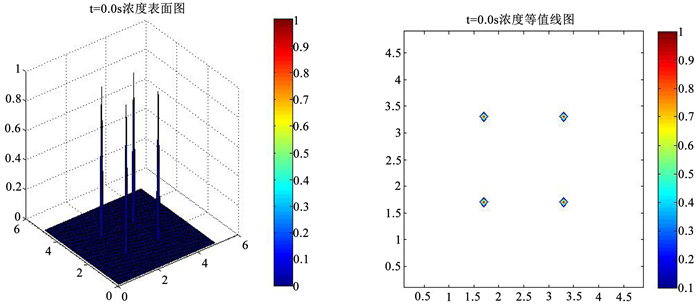Figure 1. T = 0.0 s time concentration surface map and contour map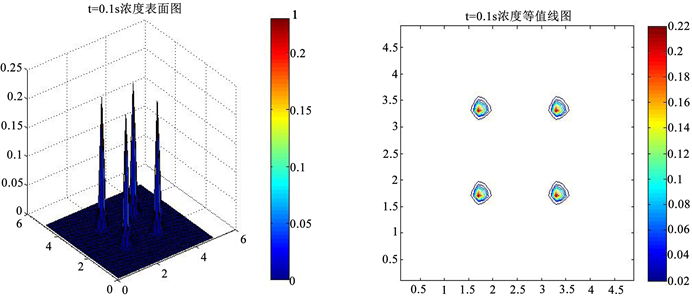Figure 2. T = 0.1 s time concentration surface map and contour map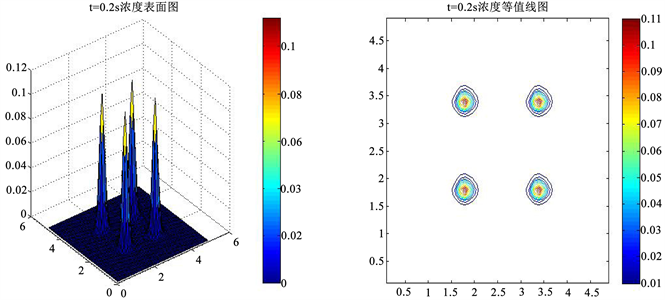Figure 3. T = 0.2 s time concentration surface map and contour map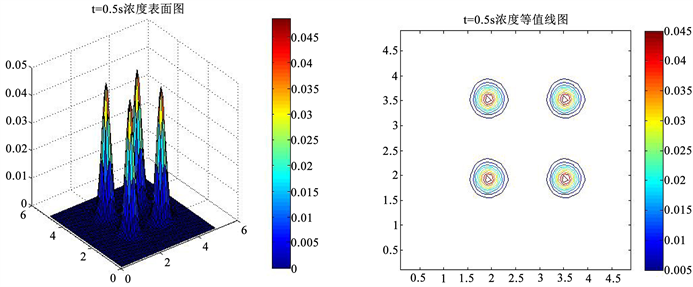Figure 4. T = 0.5 s time concentration surface map and contour map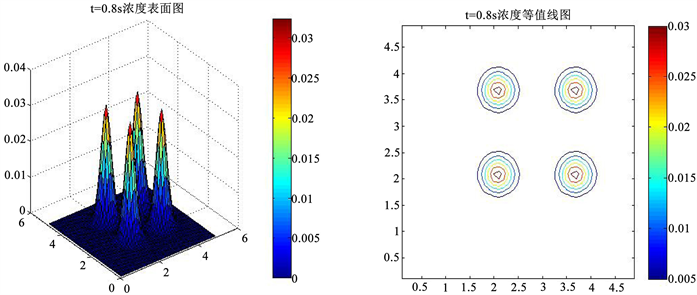Figure 5. T = 0.8 s time concentration surface map and contour map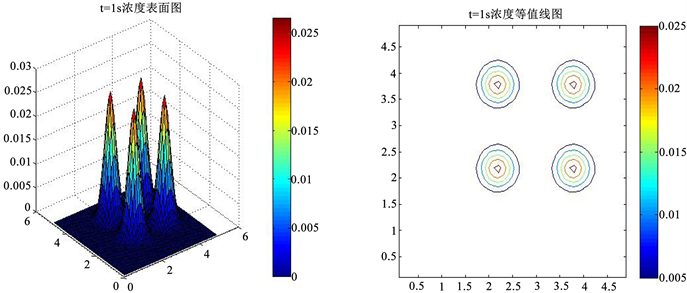Figure 6. T = 1.0 s time concentration surface map and contour map

Non Oscillation Conservative Characteristic Difference Method for Solving Convection Diffusion Equations[J]. 应用数学进展, 2018, 07(11): 1446-1457. https://doi.org/10.12677/AAM.2018.711169

1. 1. 童小红, 王兴, 苏李君, 等. 对流扩散方程的特征线EFG算法[J]. 工程数学学报, 2018, 35(2): 179-192.

2. 2. Douglas, J. and Thomas, F. (1982) Numerical Methods for Convention Dominated Diffusion Problems Based on Combining the Method of Characteristics with Finite Element or Finite Difference Procedures. SIAM Journal on Mathematic Analysis, 10, 871-885.

3. 3. Gabriel, R., Burman, E. and Karakatsani, F. (2017) Blending Low-Order Stabilised Finite Element Methods: A Positivity Preserving Local Projection Method for the Convection-Diffusion Equation. Computer Methods in Applied Mechanics and Engineering, 139, 23-26.

4. 4. 梁栋. 对流扩散方程的一类迎风格式[J]. 计算数学, 1991(2): 133-141.

5. 5. 袁益让, 梁栋, 芮洪兴, 海水入侵及防治工程的数值模拟[J]. 计算物理, 2001, 18(6): 556-562.

6. 6. Zhou, Z. and Liang, D. (2017) The Mass-Preserving and Modified-Upwind Splitting DDM Scheme for Time Dependent Convection Diffusion Equations. Journal of Computational and Applied Mathematics, 317, 247-273. https://doi.org/10.1016/j.cam.2016.10.031

7. 7. 陆金普, 刘晓遇, 杜正平. 对流占优扩散问题的一种特征差分方法[J]. 清华大学学报, 2002, 42(8): 111-113.

8. 8. 由同顺. 对流扩散方程的本质非振荡特征差分方法[J]. 应用数学, 2000, 21(4): 89-94.

9. 9. 黄素珍, 张鲁明. 对流扩散方程的一种高精度特征差分格式[J]. 南京师大学报, 2005, 28(2): 38-41.

10. 10. Douglas, J., Huang, C. and Pereira, F. (1999) The Modified Method of Characteristics with Adjust Advection. Numerische Mathematik, 83, 353-369. https://doi.org/10.1007/s002110050453

11. 11. Rui, H. and Tabata, M. (2010) A Mass-Conservative Characteristic Finite Element Scheme for Convection-Diffusion Problem. Journal of Scientific Computing, 43, 416-432. https://doi.org/10.1007/s10915-009-9283-3

12. 12. Fu, K. and Liang, D. (2016) The Conservative Characteristic FD Methods for Atmospheric Aerosol Transport Problems. Journal of Computational Physics, 305, 494-520. https://doi.org/10.1016/j.jcp.2015.10.049

13. 13. Zhou, Z. and Liang, D. (2016) The Mass-Preserving S-DDM Scheme for Two-Dimensional Parabolic Equations. Communications in Computational Physics, 19, 411-441. https://doi.org/10.4208/cicp.070814.190615a

14. 14. Zhou, Z., Liang, D. and Wong, Y. (2018) The New Mass-Conserving S-DDM Scheme for Two-Dimensional Parabolic Equations with Variable Coefficients. Applied Mathematics and Computation, 338, 882-902. https://doi.org/10.1016/j.amc.2018.06.021

15. 15. 柳忠伟. 求解高维对流扩散方程的高效本质非振荡特征差分方法[D]: [学士学位论文]. 泰安: 山东农业大学, 2017.

16. 16. 李清扬, 王能超, 易大义. 数值分析[M]. 第5版. 北京: 清华大学出版社, 2008.

17. NOTES

*通讯作者。